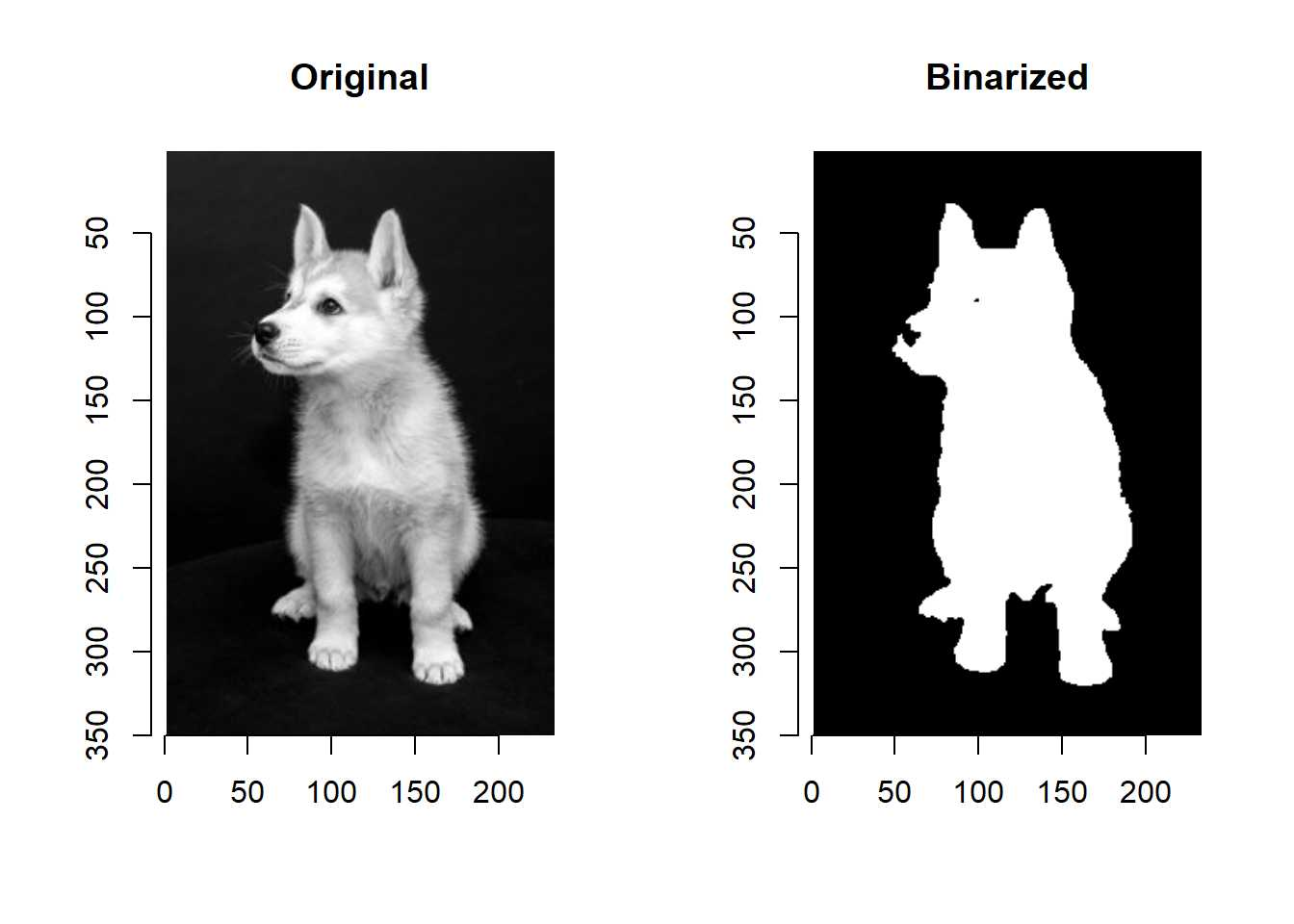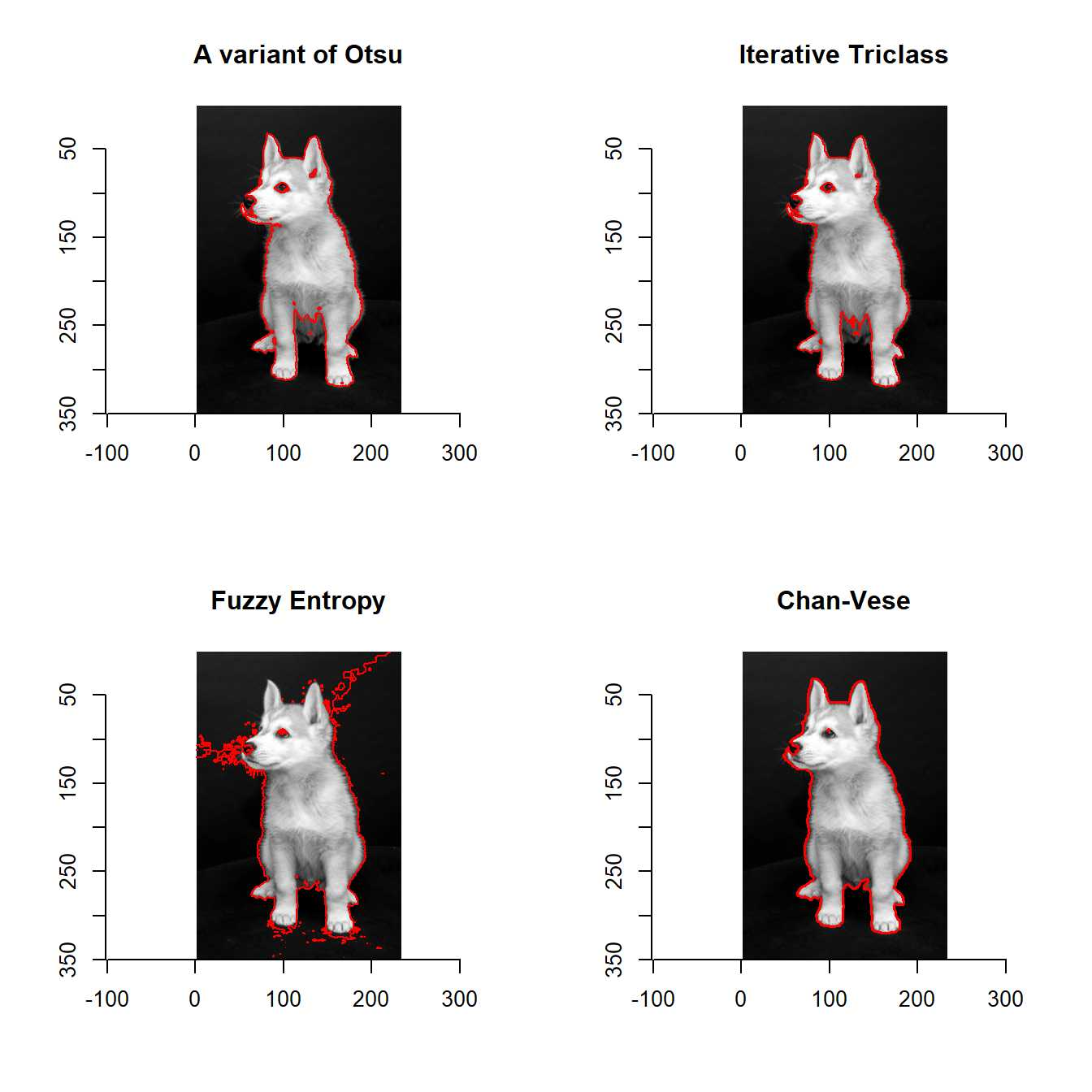Chan-Vese Segmentation

Chan-Vese segmentation is a region-based segmentation algorithm.

Region-based segmentation algorithm can extract objects whose pixesl values are not homogeneous.

I implemented Chan-Vese semgmentation (SegmentCV).

We can use the function as shown below.

devtools::install_github("ShotaOchi/imagerExtra")
library(imagerExtra)
g <- grayscale(dogs)
layout(matrix(1:2, 1, 2))
plot(g, main = "Original")
b <- SegmentCV(g, lambda2 = 15) %>% plot(main = "Binarized")We can see the belly of the dog was extracted.

It’s difficult to extract the belly if we use histogram-based segmentation.

Red curve means boundary of extracted object.

layout(matrix(1:4, 2, 2))
plot(g, main = "A variant of Otsu"); highlight(threshold(g))
plot(g, main = "Fuzzy Entropy"); highlight(ThresholdFuzzy(g))
plot(g, main = "Iterative Triclass"); highlight(ThresholdTriclass(g))
plot(g, main = "Chan-Vese"); highlight(b)